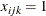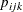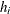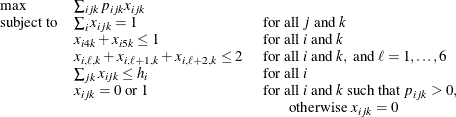# The OPTMILP Procedure

### Example 11.4 Scheduling

This example is intended for users who prefer to use the SAS DATA step, PROC SQL, and similar programming methods to prepare data for input to SAS/OR optimization procedures. SAS/OR users who prefer to use the algebraic modeling capabilities of PROC OPTMODEL to specify optimization models should consult Example 7.1 in Chapter 7: The Mixed Integer Linear Programming Solver, for a discussion of the same business problem in a PROC OPTMODEL context.

Scheduling is an application area where techniques in model generation can be valuable. Problems that involve scheduling are often solved with integer programming and are similar to assignment problems. In this example, you have eight one-hour time slots in each of five days. You have to assign four people to these time slots so that each slot is covered every day. You allow the people to specify preference data for each slot on each day. In addition, there are constraints that must be satisfied:

• Each person has some slots for which they are unavailable.

• Each person must have either slot 4 or 5 off for lunch.

• Each person can work only two time slots in a row.

• Each person can work only a specified number of hours in the week.

To formulate this problem, letdenote person,denote time slot, anddenote day. Then, letif personis assigned to time sloton day, and 0 otherwise; letdenote the preference of personfor sloton day; and letdenote the number of hours in a week that personwill work. Then, you getTo solve this problem, create a data set that has the hours and preference data for each individual, time slot, and day. A 10 represents the most desirable time slot, and a 1 represents the least desirable time slot. In addition, a 0 indicates that the time slot is not available.

data raw;
input name $hour slot mon tue wed thu fri; datalines; marc 20 1 10 10 10 10 10 marc 20 2 9 9 9 9 9 marc 20 3 8 8 8 8 8 marc 20 4 1 1 1 1 1 marc 20 5 1 1 1 1 1 marc 20 6 1 1 1 1 1 marc 20 7 1 1 1 1 1 marc 20 8 1 1 1 1 1 mike 20 1 10 9 8 7 6 mike 20 2 10 9 8 7 6 mike 20 3 10 9 8 7 6 mike 20 4 10 3 3 3 3 mike 20 5 1 1 1 1 1 mike 20 6 1 2 3 4 5 mike 20 7 1 2 3 4 5 mike 20 8 1 2 3 4 5 bill 20 1 10 10 10 10 10 bill 20 2 9 9 9 9 9 bill 20 3 8 8 8 8 8 bill 20 4 0 0 0 0 0 bill 20 5 1 1 1 1 1 bill 20 6 1 1 1 1 1 bill 20 7 1 1 1 1 1 bill 20 8 1 1 1 1 1 bob 20 1 10 9 8 7 6 bob 20 2 10 9 8 7 6 bob 20 3 10 9 8 7 6 bob 20 4 10 3 3 3 3 bob 20 5 1 1 1 1 1 bob 20 6 1 2 3 4 5 bob 20 7 1 2 3 4 5 bob 20 8 1 2 3 4 5 ;  These data are read by the following DATA step, and an integer program is built to solve the problem. The model is saved in the data set named MODEL, which is constructed in the following steps: 1. The objective function is built using the data saved in the RAW data set. 2. The constraints that ensure that no one works during a time slot during which they are unavailable are built. 3. The constraints that require a person to be working in each time slot are built. 4. The constraints that allow each person time for lunch are added. 5. The constraints that restrict people to only two consecutive hours are added. 6. The constraints that limit the time that any one person works in a week are added. 7. The constraints that allow a person to be assigned only to a time slot for which he is available are added. The statements to build each of these constraints follow the formulation closely. data model; array workweek{5} mon tue wed thu fri; array hours{4} hours1 hours2 hours3 hours4; retain hours1-hours4; set raw end=eof; length _row_$ 8 _col_ $8 _type_$ 8;
keep _type_ _col_ _row_ _coef_;

if      name='marc' then i=1;
else if name='mike' then i=2;
else if name='bill' then i=3;
else if name='bob'  then i=4;

hours{i}=hour;

/* build the objective function */

do k=1 to 5;
_col_='x'||put(i,1.)||put(slot,1.)||put(k,1.);

_row_='object';
_coef_=workweek{k} * 1000;
output;
end;

/* build the rest of the model */

/* cannot work during unavailable slots */
do k=1 to 5;
if workweek{k}=0 then do;
_row_='off'||put(i,1.)||put(slot,1.)||put(k,1.);
_type_='eq';
_col_='_RHS_';
_coef_=0;
output;
_col_='x'||put(i,1.)||put(slot,1.)||put(k,1.);
_coef_=1;
_type_=' ';
output;
end;
end;

if eof then do;
_coef_=.;
_col_=' ';

/* every hour 1 person working */
do j=1 to 8;
do k=1 to 5;
_row_='work'||put(j,1.)||put(k,1.);
_type_='eq';
_col_='_RHS_';
_coef_=1;
output;
_coef_=1;
_type_=' ';
do i=1 to 4;
_col_='x'||put(i,1.)||put(j,1.)||put(k,1.);
output;
end;
end;
end;

/* each person has a lunch */
do i=1 to 4;
do k=1 to 5;
_row_='lunch'||put(i,1.)||put(k,1.);
_type_='le';
_col_='_RHS_';
_coef_=1;
output;
_coef_=1;
_type_=' ';
_col_='x'||put(i,1.)||'4'||put(k,1.);
output;
_col_='x'||put(i,1.)||'5'||put(k,1.);
output;
end;
end;

/* work at most 2 slots in a row */
do i=1 to 4;
do k=1 to 5;
do l=1 to 6;
_row_='seq'||put(i,1.)||put(k,1.)||put(l,1.);
_type_='le';
_col_='_RHS_';
_coef_=2;
output;
_coef_=1;
_type_=' ';
do j=0 to 2;
_col_='x'||put(i,1.)||put(l+j,1.)||put(k,1.);
output;
end;
end;
end;
end;

/* work at most n hours in a week */
do i=1 to 4;
_row_='capacit'||put(i,1.);
_type_='le';
_col_='_RHS_';
_coef_=hours{i};
output;
_coef_=1;
_type_=' ';
do j=1 to 8;
do k=1 to 5;
_col_='x'||put(i,1.)||put(j,1.)||put(k,1.);
output;
end;
end;
end;
end;
run;


Next, this SAS data set is converted to an MPS-format SAS data set by establishing the structure of the MPS format and through very minor conversions of the data.

/* the following code transforms the above sparse data set */
/* into an MPS-format data set                             */

/* generate the header of the MPS-format data set */
data mps0;
format field1 field2 field3 $10.; format field4 10.; format field5$10.;
format field6 10.;
field1 = 'NAME';
field2 = '       ';
field3 = 'PROBLEM';
field4 = .;
field5 = '       ';
field6 = .;
output;
field1 = 'ROWS';
field3 = '';
output;
field1 = 'MAX';
field2 = 'object';
field3 = '';
output;
run;

/* generate rows */
proc sql;
create table mps1 as
select _type_ as field1, _row_ as field2 from model
where _row_ eq 'object' and _type_ ne '' union
select 'E' as field1, _row_ as field2 from model
where _type_ eq 'eq' union
select 'L' as field1, _row_ as field2 from model
where _type_ eq 'le' union
select 'G' as field1, _row_ as field2 from model
where _type_ eq 'ge';
quit;

/* indicate start of columns section and declare type of all */
/* variables as integer                                      */
data mps2;
format field1 field2 field3  $10.; format field4 10.; format field5$10.;
format field6 10.;
field1 = 'COLUMNS';
field2 = '         ';
field3 = '         ';
field4 = .;
field5 = '         ';
field6 = .;
output;
field1 = '         ';
field2 = '.MARK0';
field3 = "'MARKER'";
field4 = .;
field5 = "'INTORG'";
field6 = .;
output;
run;

/* generate columns */
data mps3;
set model;
format field1 field2 field3 $10.; format field4 10.; format field5$10.;
format field6 10.;
keep field1-field6;
field1 = '      ';
field2 = _col_;
field3 = _row_;
field4 = _coef_;
field5 = '      ';
field6 = .;
if field2 ne '_RHS_'  then do;
output;
end;
run;

/* sort columns by variable names */
proc sort data=mps3;
by field2;
run;

/* indicate the end of the columns section */
data mps4;
format field1 field2 field3  $10.; format field4 10.; format field5$10.;
format field6 10.;
field1 = '         ';
field2 = '.MARK1';
field3 = "'MARKER'";
field4 = .;
field5 = "'INTEND'";
field6 = .;
output;
run;

/* indicate the start of the RHS section */
data mps5;
format field1 field2 field3  $10.; format field4 10.; format field5$10.;
format field6 10.;
field1 = 'RHS';
run;

/* generate RHS entries */
data mps6;
set model;
format field1 field2 field3 $10.; format field4 10.; format field5$10.;
format field6 10.;
keep field1-field6;
field1 = '      ';
field2 = _col_;
field3 = _row_;
field4 = _coef_;
field5 = '      ';
field6 = .;
if field2 eq '_RHS_'  then do;
output;
end;
run;

/* denote the end of the MPS-format data set */
data mps7;
format field1 field2 field3  $10.; format field4 10.; format field5$10.;
format field6 10.;
field1 = 'ENDATA';
run;

/* merge all sections of the MPS-format data set */
data mps;
format field1 field2 field3 $10.; format field4 10.; format field5$10.;
format field6 10.;
set mps0 mps1 mps2 mps3 mps4 mps5 mps6 mps7;
run;


The model is solved using the OPTMILP procedure. The option PRIMALOUT=SOLUTION causes PROC OPTMILP to save the primal solution in the data set named SOLUTION.

/* solve the binary program */
proc optmilp data=mps
printlevel=0 loglevel=0
primalout=solution maxtime=1000;
run;


The following DATA step takes the solution data set SOLUTION and generates a report data set named REPORT. It restores the original interpretation (person, shift, day) of the variable names xijk so that a more meaningful report can be written. Then PROC TABULATE is used to display a schedule that shows how the eight time slots are covered for the week.

/* report the solution */
title 'Reported Solution';

data report;
set solution;
keep name slot mon tue wed thu fri;
if substr(_var_,1,1)='x' then do;
if _value_>0 then do;
n=substr(_var_,2,1);
slot=substr(_var_,3,1);
d=substr(_var_,4,1);
if      n='1' then name='marc';
else if n='2' then name='mike';
else if n='3' then name='bill';
else               name='bob';
if      d='1' then mon=1;
else if d='2' then tue=1;
else if d='3' then wed=1;
else if d='4' then thu=1;
else               fri=1;
output;
end;
end;
run;

proc format;
value xfmt 1='  xxx   ';
run;

proc tabulate data=report;
class name slot;
var mon--fri;
table (slot * name), (mon tue wed thu fri)*sum=' '*f=xfmt.
/misstext=' ';
run;


Output 11.4.1 from PROC TABULATE summarizes the schedule. Notice that the constraint that requires a person to be assigned to each possible time slot on each day is satisfied.

Output 11.4.1: A Scheduling Problem

 Reported Solution

mon tue wed thu fri
slot name   xxx xxx   xxx
1 bill
marc       xxx
mike xxx
2 bill       xxx xxx
bob xxx
marc   xxx xxx
3 bill     xxx xxx
bob xxx xxx
marc         xxx
4 bob     xxx xxx xxx
mike xxx xxx
5 bill         xxx
bob   xxx
marc xxx   xxx xxx
6 bill xxx
bob     xxx   xxx
mike   xxx   xxx
7 bob xxx xxx   xxx xxx
mike     xxx
8 bob xxx   xxx
mike   xxx   xxx xxx

Recall that PROC OPTMILP puts a character string in the macro variable _OROPTMILP_ that describes the characteristics of the solution on termination. This string can be parsed using macro functions, and the information obtained can be used in report writing. The variable can be written to the log with the following command:

%put &_OROPTMILP_;


This command produces the output shown in Output 11.4.2.

Output 11.4.2: _OROPTMILP_ Macro Variable

 STATUS=OK   ALGORITHM=BAC   SOLUTION_STATUS=OPTIMAL   OBJECTIVE=211000 RELATIVE_GAP=0   ABSOLUTE_GAP=0   PRIMAL_INFEASIBILITY=0 BOUND_INFEASIBILITY=0   INTEGER_INFEASIBILITY=0   BEST_BOUND=211000   NODES=1 ITERATIONS=71   PRESOLVE_TIME=0.02   SOLUTION_TIME=0.06

From this output you learn, for example, that at termination the solution is integer-optimal and has an objective value of 211,000.